# Texas Go Math Grade 1 Lesson 7.5 Answer Key Break Apart to Subtract

Refer to our Texas Go Math Grade 1 Answer Key Pdf to score good marks in the exams. Test yourself by practicing the problems from Texas Go Math Grade 1 Lesson 7.5 Answer Key Break Apart to Subtract.

## Texas Go Math Grade 1 Lesson 7.5 Answer Key Break Apart to Subtract

Essential Question
How do you break apart a number to subtract?
Explanation:
Suppose take an example of 15 – 6
we have break 15 as 1 and 5 to make a 10
15 – 5 = 10
10 – 1 = 9
so, 15 – 6 = 9
By this way we can easily solve to subtract

Explore
Use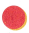to solve each problem Draw to show your work.For The Teacher

• Read the following problem. Tom had 14 pennies. He gave 4 pennies to his sister. How many pennies does Tom have now? Have children use the top workspace to solve. Then read this part of the problem: Then Tom gave 2 pennies to his brother. How many pennies does Tom have now?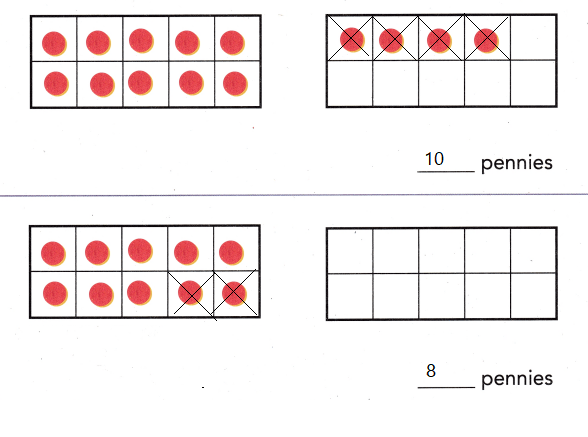Explanation:
Tom had 14 pennies. He gave 4 pennies to his sister.
14 – 4 = 10
10  pennies that Tom have now.
Then Tom gave 2 pennies to his brother.
10 – 2 = 8
8 pennies that Tom have now

Math Talk
Mathematical processes
How many pennies did Tom give away? Explain.

Model and Draw

Think about ten to find 13 — 4.
Place 13 counters in two ten frames.

How many do you subtract to get to 10?Explanation:
3 we need to subtract to get 10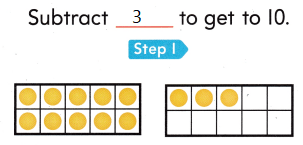How many more to subtract 4?
Then Subtract __ more.
1 more we have to subtract to get 4
Then Subtract 1 more.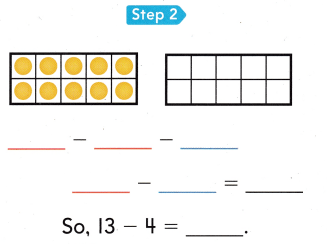Explanation: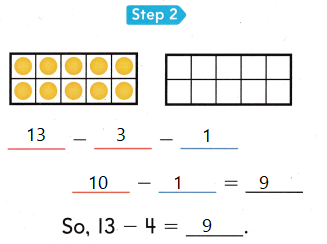Share and Show

Think
What is the best way to break apart the 7?

Subtract.

Question 1.
What is 15 – 7?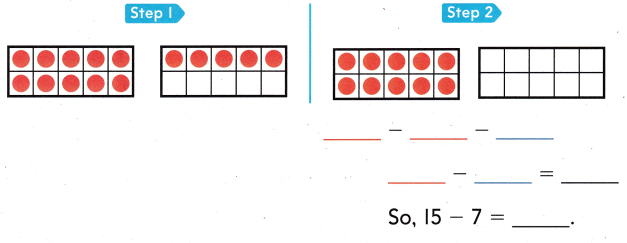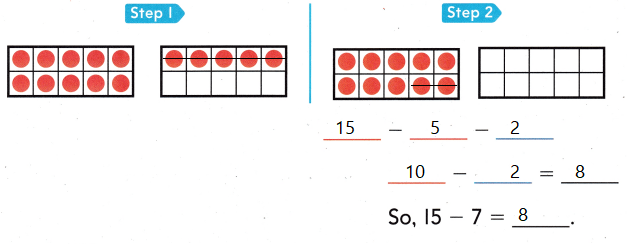Explanation:
To break a part 7 the best way is 2 and 5
15 – 5 = 10
10 – 2 = 8
15 – 7 = 8

Problem Solving

Question 2.
What is 16 – 7?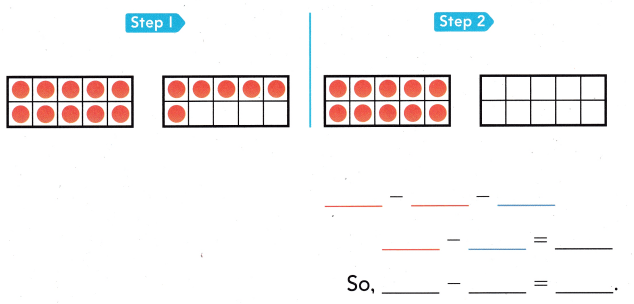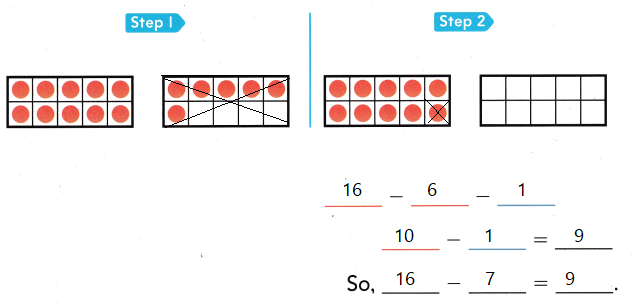Explanation:
In this problem the best way to break 7 is 6 and 1
16 – 6 = 10
10 – 1 = 9
16 – 7 = 9

Question 3.
Multi-Step There are 14 sheep in the herd. 5 sheep run away. How many sheep are left in the herd?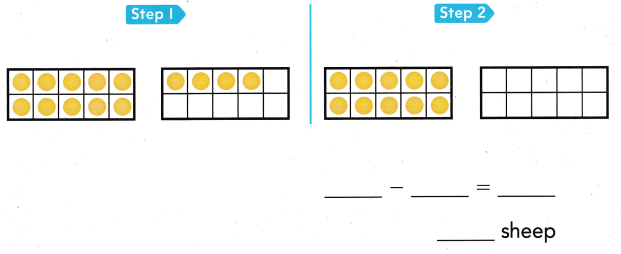Explanation:
There are 14 sheep in the herd. 5 sheep run away.
14 – 5
break 5 to make 10; 1 and 4
14 – 4 = 10
10 – 1 = 9
9 sheep are left in the herd

Question 4.
Apply Joey and Liz drew pictures of 15 items in their backyard. Joey drew 7 of the pictures. Liz drew the rest. How many pictures did Liz draw?
(A) 8
(B) 9
(C) 7
Explanation:
15 – 7
breaking 7 as 2 and 5
15 – 5 = 10
10 – 2 = 8
8 pictures that Liz draw

Question 5.
Joey has 17 rocks. He loses some. Joey now has 8 rocks. How many rocks did he lose?
(A) 9
(B) 7
(C) 8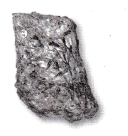Explanation:
17 – 8
Breaking 8 as 7 and 1
17 – 7 = 10
10 – 1 = 9
Joey loses 9 rocks

Question 6.
Liz picked up 13 leaves in the backyard. 5 leaves blew away. How many leaves does Liz have now?
(A) 6
(B) 5
(C) 8
Explanation:
13 – 5
Breaking 5 as 3 and 2
13 – 3 = 10
10 – 2 = 8
8 leaves that Liz have now

Question 7.
Texas Test Prep Which way shows how to make a ten to solve 15-6?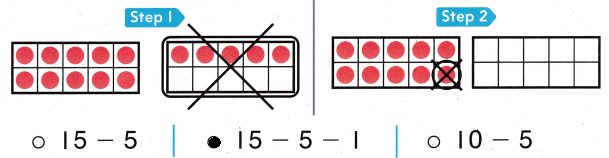Explanation:
15 – 6
Breaking 6 as 5 and 1
15- 5 = 10
10 – 1 = 9

Take Home Activity
• Tell your child o subtraction story. Ask your child to use small objects to act out the problem to solve it.

### Texas Go Math Grade 1 Lesson 7.5 Homework and Practice Answer Key

Question 1.
What is 14 – 6 = ?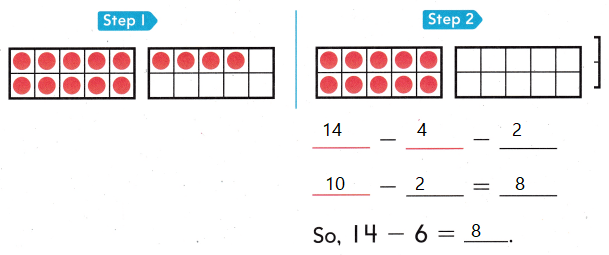Explanation:
14 – 6
Breaking 6 as 4 and 2
14 – 4 = 10
10 – 2 = 8

Problem Solving

Question 2.
Multi-Step Alex saw 15 monkeys in a tree. 6 went to look for food. How many monkeys are in the tree?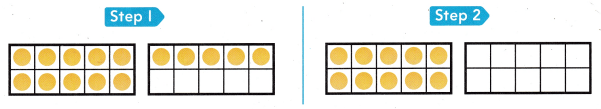___ – ___ = ____
___ monkeys
15 – 6 = 9
9 monkeys
Explanation:
Alex saw 15 monkeys in a tree. 6 went to look for food.
15 – 6
Breaking 6 as 5 and 1
15 – 5 = 10
10 – 1 = 9
9 monkeys are in the tree

Texas Test Prep

Lesson Check

Question 3.
Jack and Ian cut up 16 pieces of fruit to make a fruit salad. Ian cut up 7 pieces of fruit. Jack cut up the rest. How many pieces of fruit did Jack cut up?(A) 8
(B) 9
(C) 7
Explanation:
16 – 7
Breaking 7 as 6 and 1
16 – 6 = 10
10 – 1 = 9 pieces of fruit that Jack cut up

Question 4.
Maddie has 13 shells. She uses 4 to decorate a poster. How many shells does Maddie have now?
(A) 8
(B) 7
(C) 9
Explanation:
13 – 4
Breaking 4 as 3 and 1
13 – 3 = 10
10 – 1 = 9 shells that Maddie have now

Question 5.
Which way shows how to make a ten to solve 17 — 8?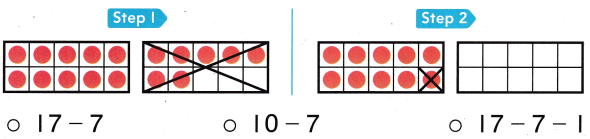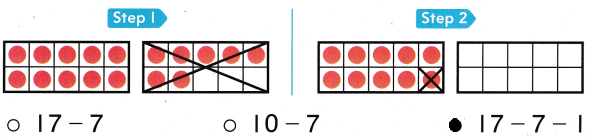Explanation:
17 – 8
Breaking 8 as 7 and 1
17 – 7 = 10
10 – 1 = 9

Question 6.
Multi-Step Addison had 16 stickers. She used 8 of them. Which way shows how to make a ten to solve the problem?